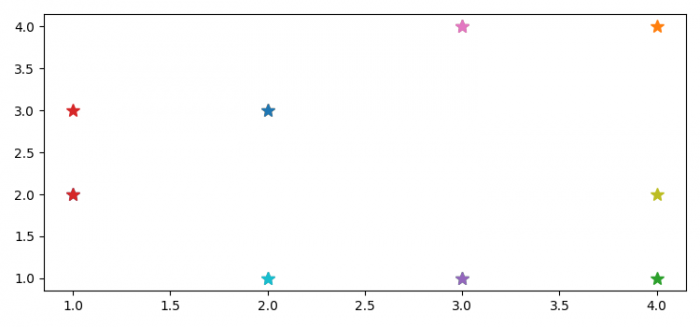# How do I redraw an image using Python's Matplotlib?

PythonServer Side ProgrammingProgramming

To redraw an image using python's Matplotlib, we can take the following steps −

• Set the figure size and adjust the padding between and around the subplots.
• Create a new figure or activate an existing figure.
• Get the current axis using gca() method.
• Show the current figure.
• Iterate in the range of 20 and redraw the plot.
• Use plot() method to plot random data points.
• Redraw on the figure and pause for a while.
• Close a figure window.

## Example

import numpy as np
from matplotlib import pyplot as plt

plt.rcParams["figure.figsize"] = [7.50, 3.50]
plt.rcParams["figure.autolayout"] = True

fig = plt.figure()
ax = fig.gca()
fig.show()

for i in range(20):
ax.plot(np.random.randint(1, 5), np.random.randint(1, 5), '*', ms=10)
fig.canvas.draw()
plt.pause(0.1)

plt.close(fig)

## Output